This video was brought to you by GeneralPAC.com, making power systems Intuitive, Open and Free for Everyone, Everywhere. Consider subscribing and supporting through patreon.com/GeneralPAC. This is a mechanism for you to support us financially so we can continue making high quality power system video tutorials. Our corporate sponsor for this topic is AllumiaX.com from Seattle, Washington. Contact them for industrial and commercial power system studies.

Fault analysis in power systems Part 4a. Reflecting fault current and voltage values on the high voltage side of the transformer.

This is the first video in Part 4 in the series on fault analysis in power systems.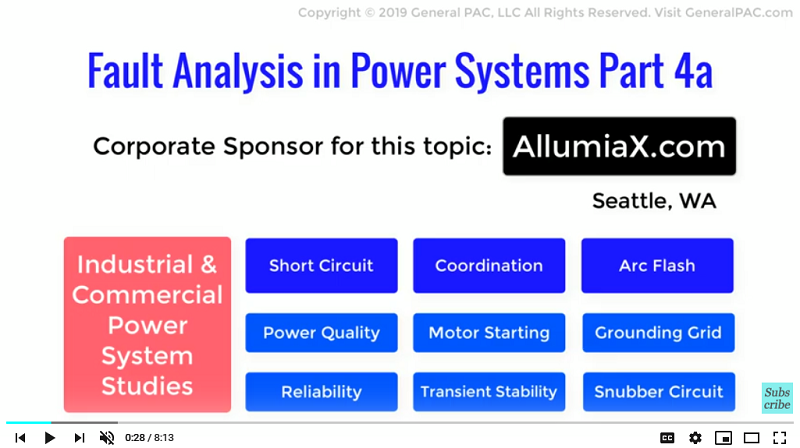In this video we will see how the faults are analyzed from the high voltage side as opposed to the low voltage side of the transformer which we have done in previous videos. This is step 7 in our fault analysis methodology, which was discussed in Part 2a and serves as an additional bonus concept.

Up untill now, we have been seeing calculation and methodologies on faults on the Low voltage side or the 13.8 kV of transformer in our examples, but let’s suppose if the fault is still on the 13.8 kV side of the bus but we need to know what the same fault current and voltage values are however, this time, seen through the HV side of the transformer or the 115 kVside of the bus. This is useful for many instances in power system protection and analysis. Because of this usefulness we will reflect these Fault current and voltage quantities of the low voltage side towards the High voltage side.

To do this, we will have to account for two changes in the sequence current values, The first one of them is Magnitude --- due to the change in voltage levels.

The second involves the Angle --- since we have a delta wye transformer angles will also be subject to change.

Units systems, which we viewed in the Introduction to Per Units series by GeneralPAC – see the description section below for a link.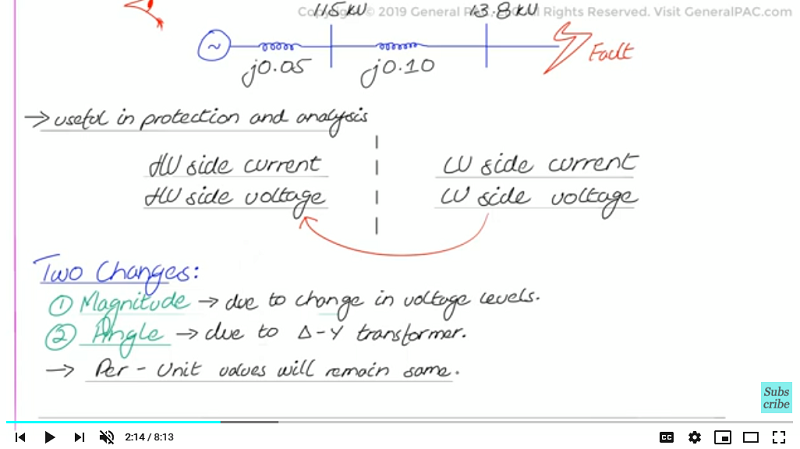Now, to account for the change in magnitude as a result of analyzing the fault on the HV side of the transformer, we simply change the base value of voltage from 13.8 kV to 115 kV. Now this allows us to convert the sequence per unit values of currents calculated earlier to the sequence ampere values reflected on the 115kV side.

The table summarizes the change in magnitude concept.

On the high voltage side the base current would equal to the base value of power divided by the square root of 3 times 115 kV and that essentially equals the base value of current on the high voltage side.

On the low voltage side however we simply take the base value of power divide that by the square root of 3 times 13.8 kV voltage magnitude. And that equals the base value of current for the low voltage side.

For the high voltage side and the low voltage side we essentially calculate the ampere values by multiplying the per unit current by the low voltage or the high voltage value.

And by utilizing the right formulas we can simply calculate the ampere, either high voltage current or the low voltage current. Now, the positive sequence values are defined in the summary table. However a similar procedure can be performed for the negative sequence component or the negative sequence value.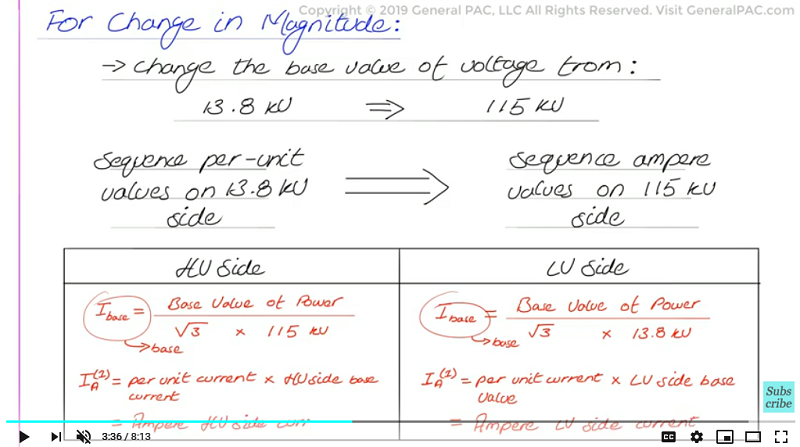Since the transformer is a delta wye transformer there will be no zero sequence current on the HV side because the delta winding traps the zero sequence current within itself. This is quite evident with the Zero Sequence network diagram as shown. We will certainly see, zero sequence current flow on the LV side however, on the HV side, zero sequence current is essentially zero for all fault types involving ground. Now, this was discussed in great detail in Part 2c of this series and you can check out the description section below for a link to that video.

Up till now, we have been talking about magnitude adjustments for positive, negative, and zero sequence currents which we know is nonexistent on the high voltage side. Now, we will shift our gear and consider the second factor which is the angle adjustment.

Because we have a very specific Delta Wye transformer connection where the LV Line current lags the HV line current by 30 degrees, or another way of saying this that we have a Dy1 or a standard D-AB Delta Wye transformer connection, because of the delta-Wye transformer connection and the difference in angles between the line current on the high voltage side and the line current on the low voltage side, we need to consider this transformation when we are reflecting the LV positive sequence and the LV negative sequence current both of them on the HV side. Now, please watch our highly in-depth tutorial on Introduction to Delta Wye Transformer to get a better understanding by what we mean by saying Dy1 or D-AB Transformer connection.

Now, in the figure below, we clearly see that the LV positive sequence line current lags the HV positive sequence line current by 30 degrees. This makes sense because we know that positive sequence current follows the same rotation as the system phasors. So when we are reflecting the LV sequence line current on the HV side, we need to advance by 30 degrees which can be accomplished by multiplying the LV per unit line current value by 1∠30 degrees (again this is to calculate the HV positive sequence line current value in per units). Keep in mind that if we had a different type of Delta Wye transformer connection, then we would have to adjust the phase angle relationship accordingly.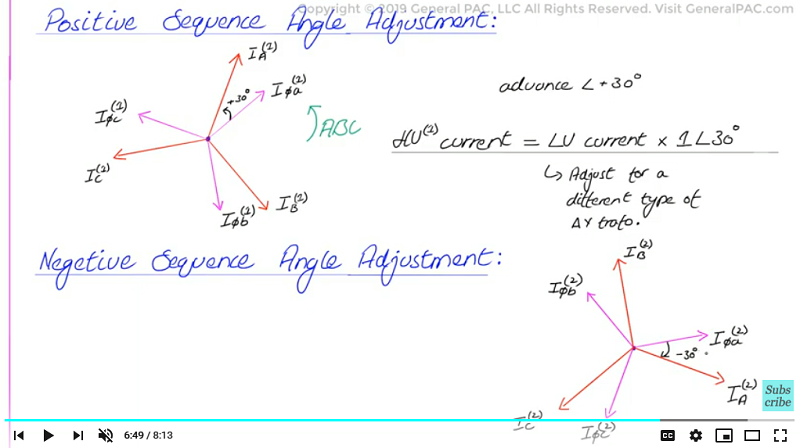For the negative sequence current, In the figure below, we clearly see that the LV negative sequence line current LEADS the HV negative sequence line current by 30 degrees. Now, this makes sense because we know that negative sequence current effectively follows in the reverse rotation as the system phasors. So when we are reflecting the LV positive sequence line current on the HV side, we need to delay by 30 degrees which can be accomplished by multiplying the LV negative sequence current in per unit values by 1∠-30 degrees. (again, this is to calculate HV negative sequence line current value in per units).

Now that we understand how to adjust values of sequence component currents, provided that we have a very specific Delta Wye transformer connection (in our case a Dy1 or a DAB), we can now move forward with reflecting the fault currents on the HV side for any given fault on the LV side of the transformer. And then from there, we can easily calculate voltage quantities as well although they are a bit more complicated.

In the next video we will show you how to put this into effect in a calculation examples for a specific type of fault on the low voltage side.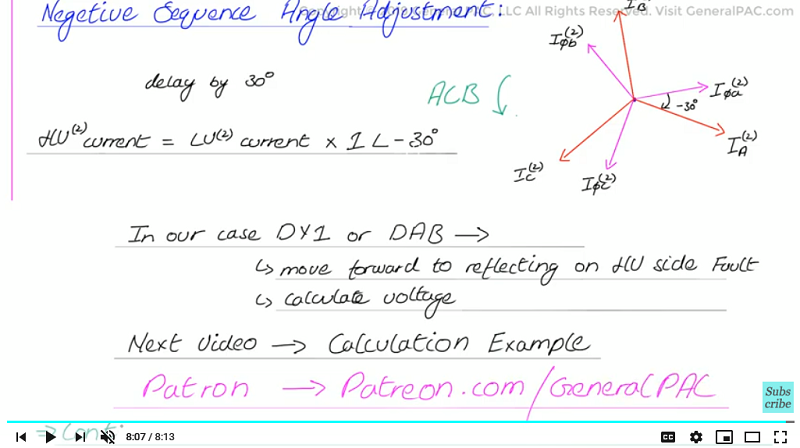Our coorporate sponsor AllumiaX,LLC published a new blog on "Fault Analysis in Power Systems". This blog covers the following topics:
• Types of Faults in Power System
• Steps to perform a Fault Analysis in a Power System
• Causes of Power System Faults#### Greetings from the GeneralPAC Team!

We make high-quality Power Systems Video Tutorials on complex topics that are free and open to everyone!  Thank you so much for supporting us through Patreon so we can continue doing good and valuable work.

What is Patreon and why do we use it?

Patreon is a fantastic portal that allows our fans and community to make monthly contribution (like Netflix subscription) so we can continue creating high-quality power systems video tutorials. In return, you get access to incredible perks like voting on future topics, getting your questions answered, access to VIP Q/A webinars with the creators of GeneralPAC, and much more! We THANK YOU for supporting us

Why do we need your support?

An incredible amount of time and effort is needed to develop high-quality video tutorials. Each video (Part 1 for example) takes approximately 10 hours to complete which includes learning the concept ourselves, brainstorming creative ways to teach and explain the concepts, writing the script, audio recording, video recording, and editing. It's no wonder why Hundreds-of-Thousands of people have watched, liked, subscribed, and left positive comments on Youtube channel. Your support truly makes all the difference.